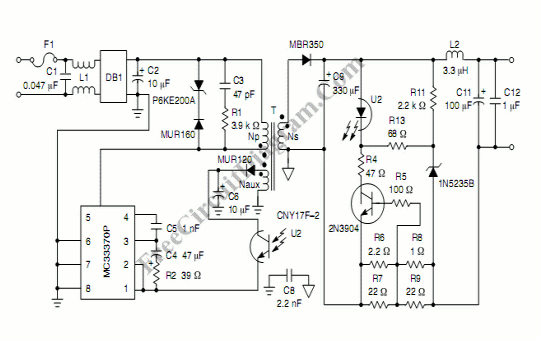# Constant Current Constant Voltage SMPS (Switch Mode Power Supply)This constant current constant voltage  SMPS can be used to efficiently charge a battery. This circuit gives 7.2V at constant voltage mode, and 600 mA at constant current mode. Here is the schematic diagram of the circuit:The mechanism is simple, when the load impedance is high, then the output voltage will be constant at 7.2V.  When the load impedance get lower then the output current will increase. Any load impedance doest affect the output voltage, the voltage will be constant, as long as the load draws no more than 600 mA current (12 ohm load). When the load trying to get more current than 600 mA (the impedance falls below 12 ohm) then the constant current mode will work. At this mode, the current will be constant. If the load impedance get lower than 12 ohm then the output voltage will drop below 7.2 volt to keep the current constant at 600 mA.

Inductor, L1:

22 mH (Siemens) Common–mode choke

Transformer:

Core Type: E13/7/4
Ferrite Material: N67 from Siemens or equivalent
Bobbin: B66202 from Siemens or equivalent
Primary: 133 turns of #35 AWG, three layers 0.002” mylar tape
Auxiliary: 27 turns of #38 AWG, two layers 0.002” mylar tape
Secondary: 8 turns of #26 AWG, triple insulated wire
Gap: 0.137 mm for a primary inductance of 2.002 mH

[Schematic diagram source: ON Semiconductor Application Notes]